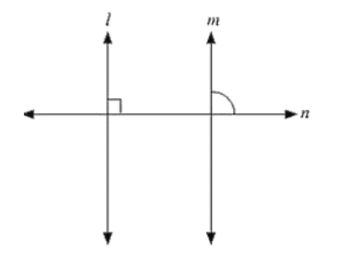# If I, m, n are three lines such that I∥ m and n perpendicular to l, prove that n perpendicular to m.

Question:

If I, m, n are three lines such that I∥ m and n perpendicular to l, prove that n perpendicular to m.Solution:

Given, l ∥ m, n perpendicular to I

To prove: n perpendicular to m

Since l ∥ m and n intersects

∴ ∠1 = ∠2             [Corresponding angles]

But, U = 90

⟹ ∠2 = 90°

Hence n is perpendicular to m